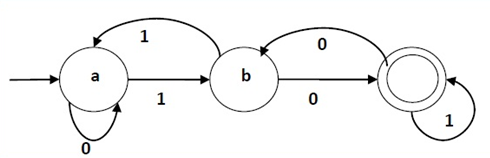# Deterministic Finite Automata (DFA)

Here, we are going to learn about the Deterministic Finite Automata (DFA): its definition, state diagram, transition function, Operation of deterministic finite automata, etc.
Submitted by Mahak Jain, on November 12, 2018

In Deterministic Finite Automata (DFA), for a given input symbol the machine will move to which next state can be determined and hence it is named as deterministic automata. The number of states is finite in number and hence it is called finite automata. Combining both the characteristics it is called DFA or deterministic finite automata.

Definition of a DFA in formal terms:

A DFA can be denoted by a 5-tuple (Q, ∑, δ, q0, F) where,

• Q is a set of states. The number of states are finite.
• is a set of symbols called the alphabet. They are finite in number.
• δ is the transition function. Here it maps Q × ∑ → Q
• q0 is the initial state or the start state from where any input is first given or is being processed (q0 ∈ Q).
• F is a set of final state/states of Q (F ⊆ Q). in other words it is a set of accepting states.

State diagram of a DFA:

• The states are denoted by vertices or circles.
• The transition is denoted by arcs which means arrows connecting one state to another.
• The initial state is denoted by an incoming arc.
• The final or the accepting state is denoted by double or two circles.

Example:

DFA:

• Q = {a, b, z},
• ∑ = {0, 1},
• q0 = {a},
• F = {z},

Transition function:

Current StateInput 0Input 1
Aab
Bza
Zbz

State diagram:What is a trap state in DFA?

If a transition leads to a state from which it can never escape. such a state is called a trap state.it is called as the dead state. There is no way of reaching the final or the accepting state from this trap or dead state.

Operation of deterministic finite automata:

Initially, it is assumed to be in an initial state or start state q0, with its input process or mechanism on the leftmost symbol of the input string. During every move of the finite automata the input tape read mechanism shifts one position to the right, so each move consumes one input symbol. At the last of the string, it is accepted if the automata are in one of the final states otherwise is rejected. The input process or mechanism can only move from left to right and not in reverse direction i.e. right to left. Also, it can only read one symbol at one time and at each step. The transition function governs the transition from one state to other.

Applications od DFA:

• In almost all compilers DFA understanding is required
• It is used in language processing systems
• Used for applications that accept typed command
• Text processors also apply the DFA basic
• It is applied is speech processing systems
• It is needed in signal processing systems
• Controllers apply the properties of DFA
• In tracking systems
• In text filters

What's New

Top Interview Coding Problems/Challenges!

Languages: » C » C++ » C++ STL » Java » Data Structure » C#.Net » Android » Kotlin » SQL
Web Technologies: » PHP » Python » JavaScript » CSS » Ajax » Node.js » Web programming/HTML
Solved programs: » C » C++ » DS » Java » C#
Aptitude que. & ans.: » C » C++ » Java » DBMS
Interview que. & ans.: » C » Embedded C » Java » SEO » HR
CS Subjects: » CS Basics » O.S. » Networks » DBMS » Embedded Systems » Cloud Computing
» Machine learning » CS Organizations » Linux » DOS
More: » Articles » Puzzles » News/Updates